# RS Aggarwal Solutions for Class 8 Chapter 13 - Time and Work Exercise 13A

Students can refer and download RS Aggarwal Solutions for Class 8 Maths Chapter 13- Exercise 13A, Time and Work from the links provided below. Our experts have solved the RS Aggarwal Solutions to help students in their exam preparation. In Exercise 13A of RS Aggarwal Class 8 Maths, we shall see problems based on Pipes and Cistern.

## Download PDF of RS Aggarwal Solutions for Class 8 Maths Chapter 13- Exercise 13A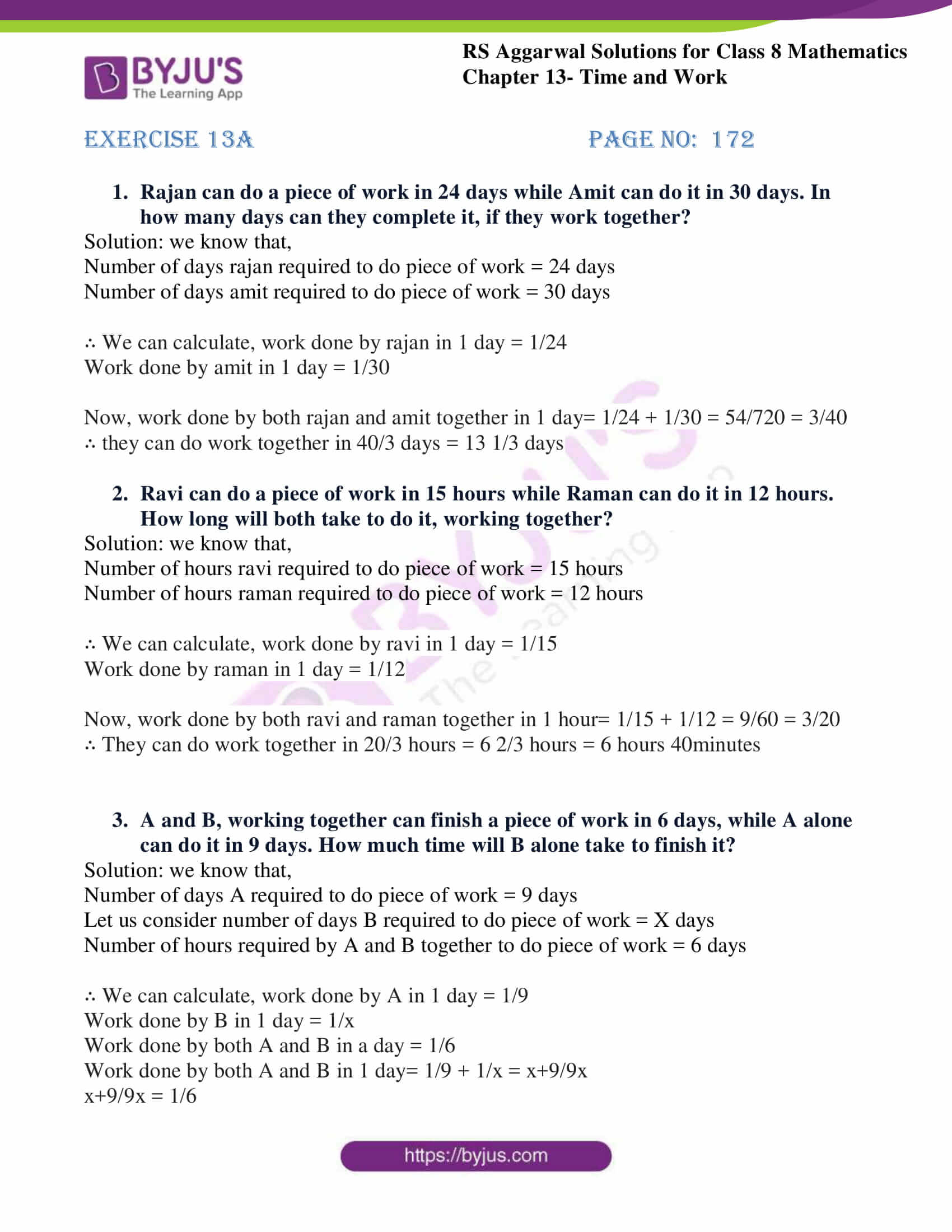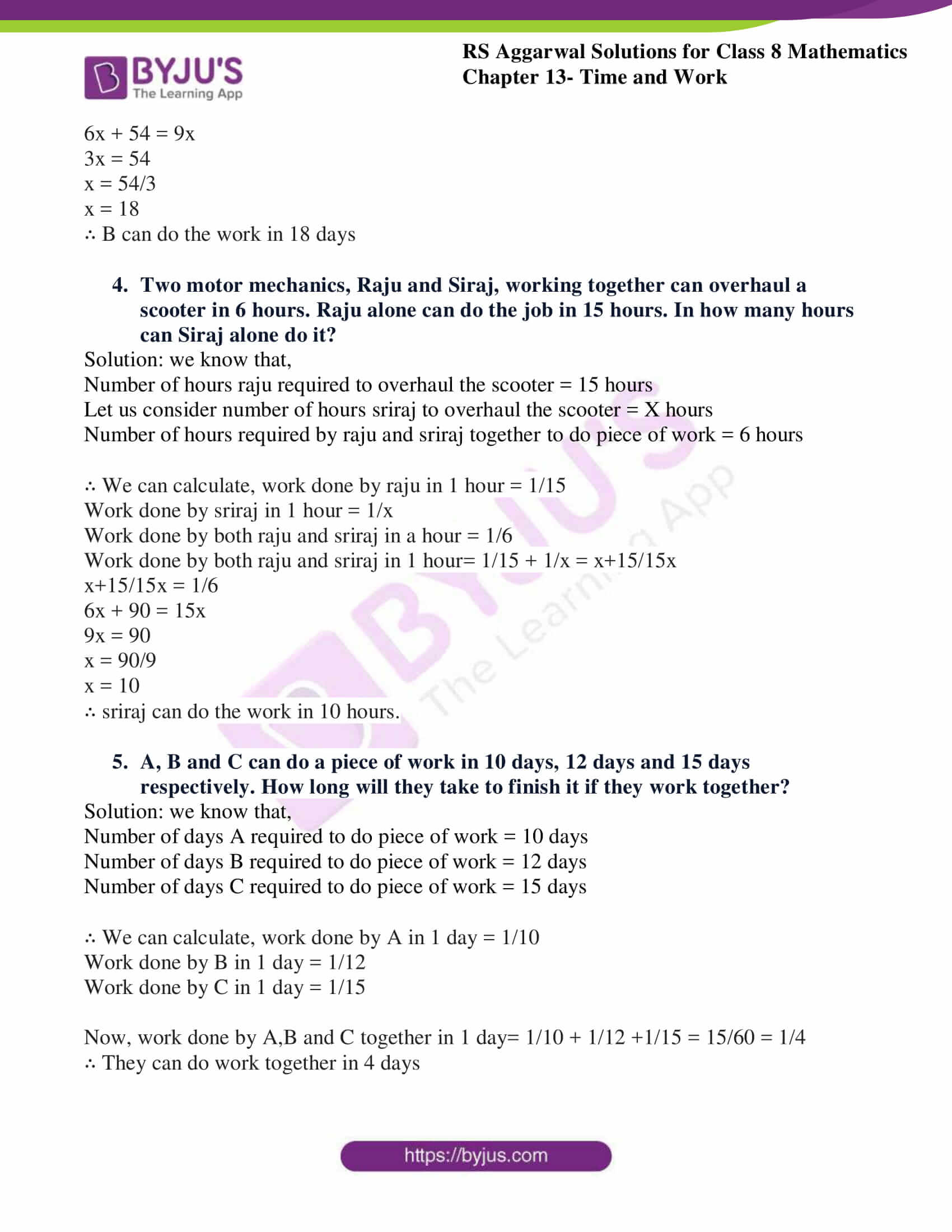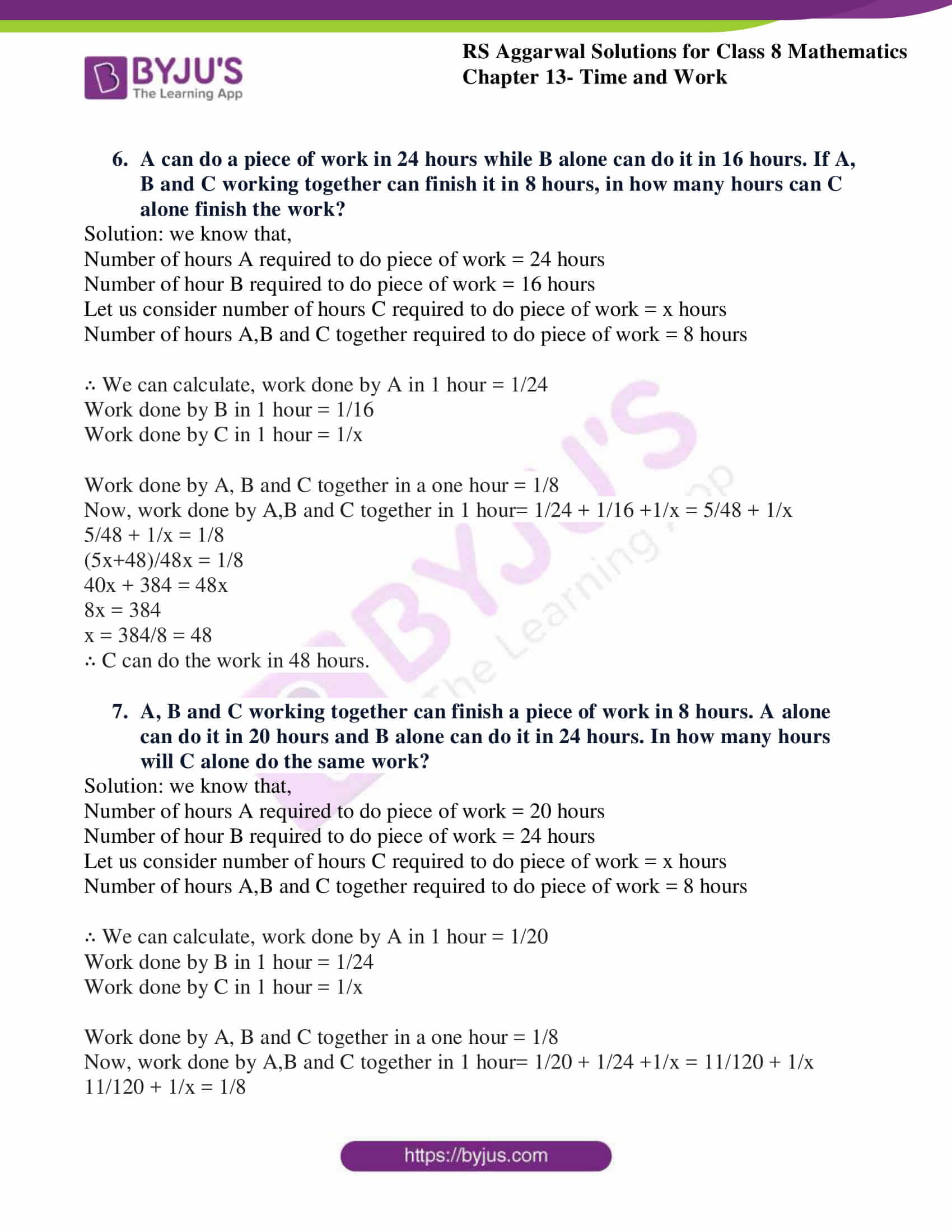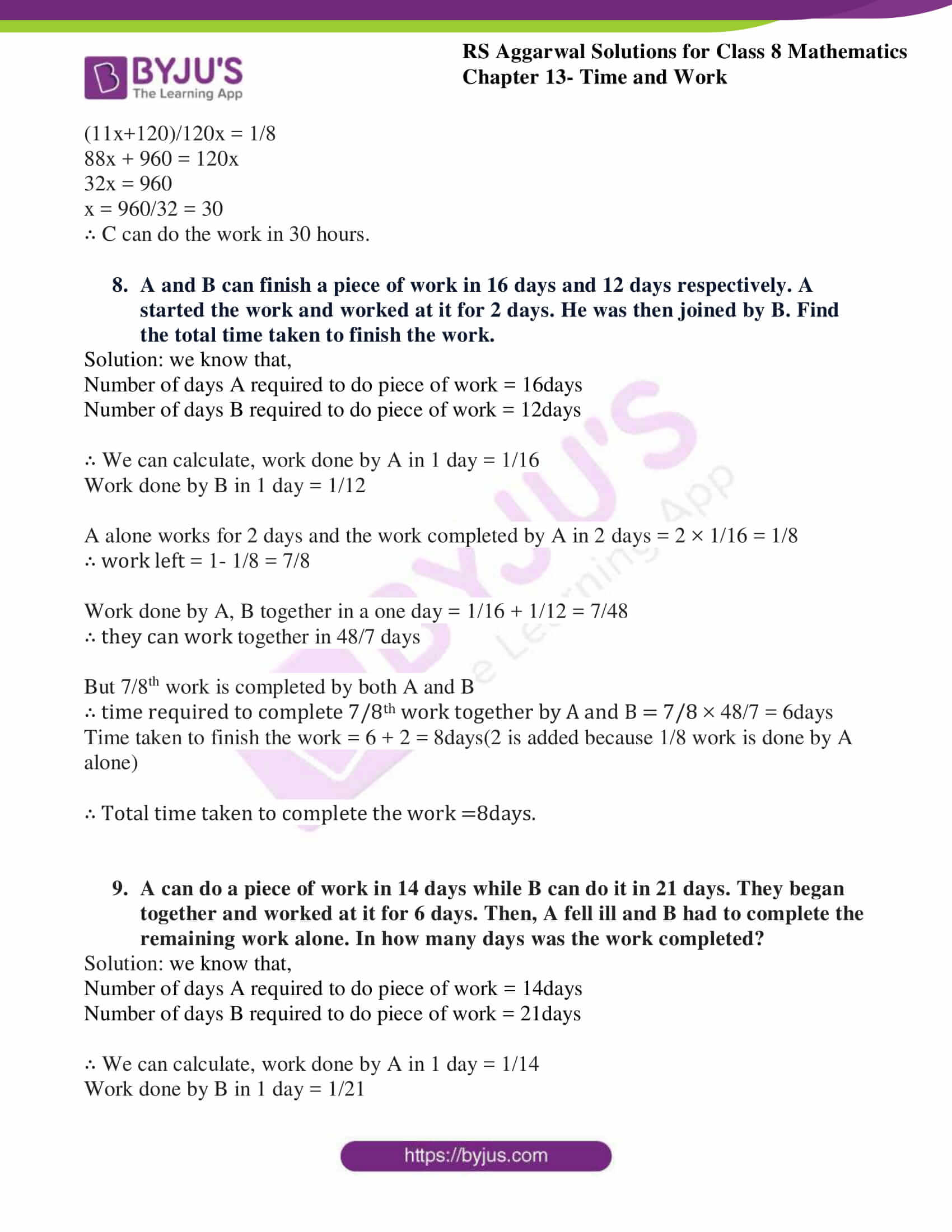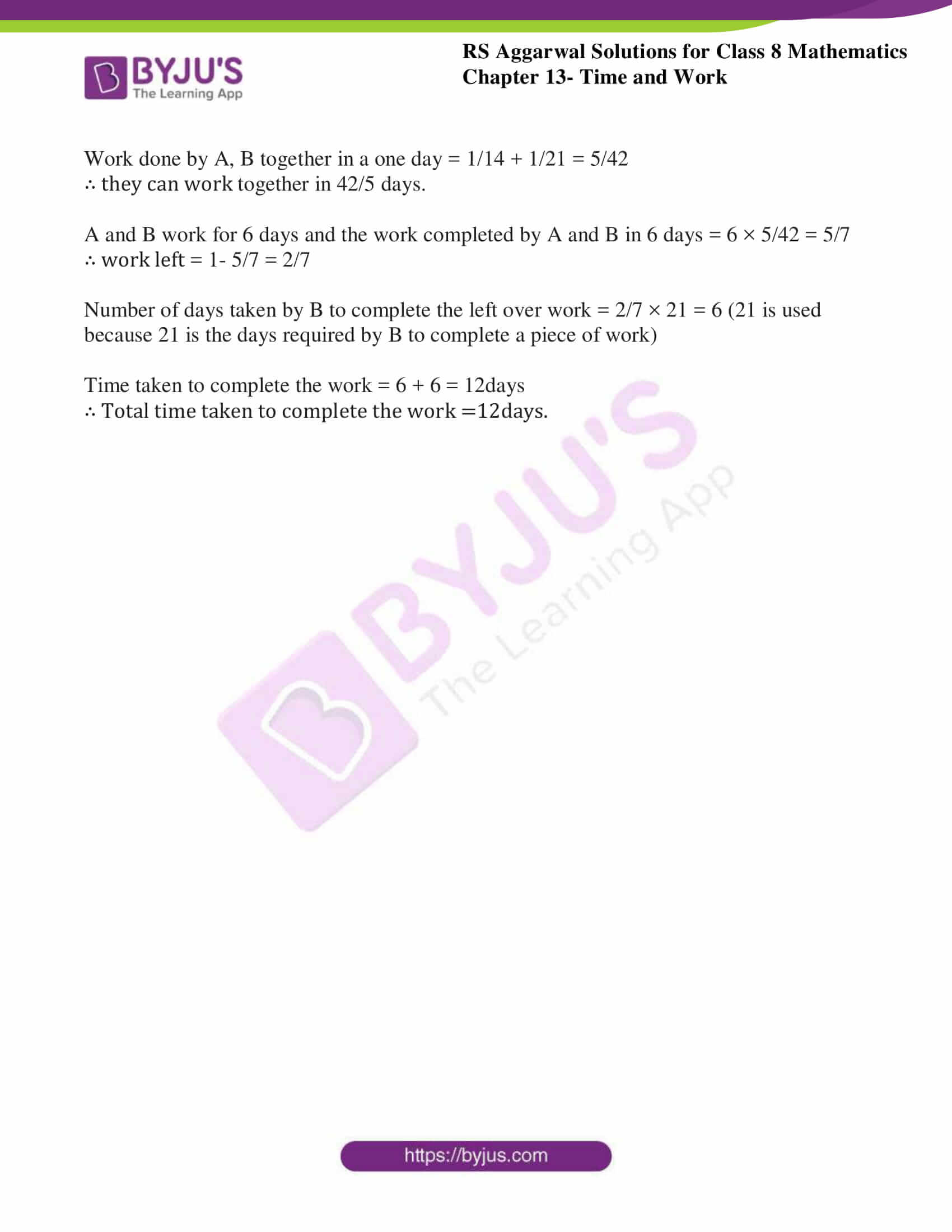### Access Answers to RS Aggarwal Solutions for Class 8 Maths Chapter 13- Time and Work Exercise 13A

1. Rajan can do a piece of work in 24 days while Amit can do it in 30 days. In how many days can they complete it, if they work together?

Solution: we know that,

Number of days Rajan required to do piece of work = 24 days

Number of days Amit required to do piece of work = 30 days

∴ We can calculate, work done by Rajan in 1 day = 1/24

Work done by Amit in 1 day = 1/30

Now, work done by both Rajan and Amit together in 1 day= 1/24 + 1/30 = 54/720 = 3/40

∴ they can do work together in 40/3 days = 13 1/3 days

2. Ravi can do a piece of work in 15 hours while Raman can do it in 12 hours. How long will both take to do it, working together?

Solution: we know that,

Number of hours Ravi required to do piece of work = 15 hours

Number of hours Raman required to do piece of work = 12 hours

∴ We can calculate, work done by Ravi in 1 day = 1/15

Work done by Raman in 1 day = 1/12

Now, work done by both Ravi and Raman together in 1 hour= 1/15 + 1/12 = 9/60 = 3/20

∴ They can do work together in 20/3 hours = 6 2/3 hours = 6 hours 40minutes

3. A and B, working together can finish a piece of work in 6 days, while A alone can do it in 9 days. How much time will B alone take to finish it?

Solution: we know that,

Number of days A required to do piece of work = 9 days

Let us consider number of days B required to do piece of work = X days

Number of hours required by A and B together to do piece of work = 6 days

∴ We can calculate, work done by A in 1 day = 1/9

Work done by B in 1 day = 1/x

Work done by both A and B in a day = 1/6

Work done by both A and B in 1 day= 1/9 + 1/x = x+9/9x

x+9/9x = 1/6

6x + 54 = 9x

3x = 54

x = 54/3

x = 18

∴ B can do the work in 18 days

4. Two motor mechanics, Raju and Siraj, working together can overhaul a scooter in 6 hours. Raju alone can do the job in 15 hours. In how many hours can Siraj alone do it?

Solution: we know that,

Number of hours Raju required to overhaul the scooter = 15 hours

Let us consider number of hours Sriraj to overhaul the scooter = X hours

Number of hours required by Raju and Sriraj together to do piece of work = 6 hours

∴ We can calculate, work done by Raju in 1 hour = 1/15

Work done by Sriraj in 1 hour = 1/x

Work done by both Raju and Sriraj in a hour = 1/6

Work done by both Raju and Sriraj in 1 hour= 1/15 + 1/x = x+15/15x

x+15/15x = 1/6

6x + 90 = 15x

9x = 90

x = 90/9

x = 10

∴ Sriraj can do the work in 10 hours.

5. A, B and C can do a piece of work in 10 days, 12 days and 15 days respectively. How long will they take to finish it if they work together?

Solution: we know that,

Number of days A required to do piece of work = 10 days

Number of days B required to do piece of work = 12 days

Number of days C required to do piece of work = 15 days

∴ We can calculate, work done by A in 1 day = 1/10

Work done by B in 1 day = 1/12

Work done by C in 1 day = 1/15

Now, work done by A,B and C together in 1 day= 1/10 + 1/12 +1/15 = 15/60 = 1/4

∴ They can do work together in 4 days

6. A can do a piece of work in 24 hours while B alone can do it in 16 hours. If A, B and C working together can finish it in 8 hours, in how many hours can C alone finish the work?

Solution: we know that,

Number of hours A required to do piece of work = 24 hours

Number of hour B required to do piece of work = 16 hours

Let us consider number of hours C required to do piece of work = x hours

Number of hours A,B and C together required to do piece of work = 8 hours

∴ We can calculate, work done by A in 1 hour = 1/24

Work done by B in 1 hour = 1/16

Work done by C in 1 hour = 1/x

Work done by A, B and C together in a one hour = 1/8

Now, work done by A,B and C together in 1 hour= 1/24 + 1/16 +1/x = 5/48 + 1/x

5/48 + 1/x = 1/8

(5x+48)/48x = 1/8

40x + 384 = 48x

8x = 384

x = 384/8 = 48

∴ C can do the work in 48 hours.

7. A, B and C working together can finish a piece of work in 8 hours. A alone can do it in 20 hours and B alone can do it in 24 hours. In how many hours will C alone do the same work?

Solution: we know that,

Number of hours A required to do piece of work = 20 hours

Number of hour B required to do piece of work = 24 hours

Let us consider number of hours C required to do piece of work = x hours

Number of hours A,B and C together required to do piece of work = 8 hours

∴ We can calculate, work done by A in 1 hour = 1/20

Work done by B in 1 hour = 1/24

Work done by C in 1 hour = 1/x

Work done by A, B and C together in a one hour = 1/8

Now, work done by A,B and C together in 1 hour= 1/20 + 1/24 +1/x = 11/120 + 1/x

11/120 + 1/x = 1/8

(11x+120)/120x = 1/8

88x + 960 = 120x

32x = 960

x = 960/32 = 30

∴ C can do the work in 30 hours.

8. A and B can finish a piece of work in 16 days and 12 days respectively. A started the work and worked at it for 2 days. He was then joined by B. Find the total time taken to finish the work.

Solution: we know that,

Number of days A required to do piece of work = 16 days

Number of days B required to do piece of work = 12 days

∴ We can calculate, work done by A in 1 day = 1/16

Work done by B in 1 day = 1/12

A alone works for 2 days and the work completed by A in 2 days = 2 × 1/16 = 1/8

∴ work left = 1- 1/8 = 7/8

Work done by A, B together in a one day = 1/16 + 1/12 = 7/48

∴ they can work together in 48/7 days

But 7/8th work is completed by both A and B

∴ time required to complete 7/8th work together by A and B = 7/8 × 48/7 = 6days

Time taken to finish the work = 6 + 2 = 8days(2 is added because 1/8 work is done by A alone)

∴ Total time taken to complete the work =8days.

9. A can do a piece of work in 14 days while B can do it in 21 days. They began together and worked at it for 6 days. Then, A fell ill and B had to complete the remaining work alone. In how many days was the work completed?

Solution: we know that,

Number of days A required to do piece of work = 14 days

Number of days B required to do piece of work = 21 days

∴ We can calculate, work done by A in 1 day = 1/14

Work done by B in 1 day = 1/21

Work done by A, B together in a one day = 1/14 + 1/21 = 5/42

∴ they can work together in 42/5 days.

A and B work for 6 days and the work completed by A and B in 6 days = 6 × 5/42 = 5/7

∴ work left = 1- 5/7 = 2/7

Number of days taken by B to complete the left over work = 2/7 × 21 = 6 (21 is used because 21 is the days required by B to complete a piece of work)

Time taken to complete the work = 6 + 6 = 12days

∴ Total time taken to complete the work =12days.

## RS Aggarwal Solutions for Class 8 Maths Chapter 13- Exercise 13A

Exercise 13A of RS Aggarwal Class 8, Time and Work. This exercise mainly deals with Pipes and Cistern problems using a few general rules.

Students are suggested to practice the problems on a regular basis which will help them excel in their exams and increase their overall percentage. Practicing as many times as possible helps in building time management skills and also boosts the confidence level to achieve high marks.